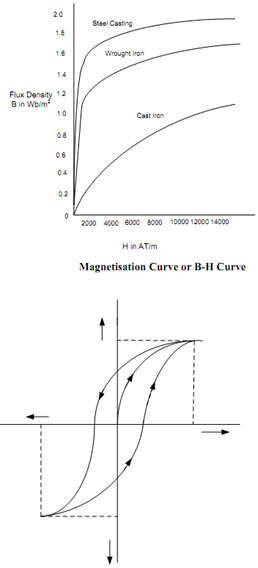## Magnetic Hysteresis Assignment Help

Assignment Help: >> ElectroMagnetism - Magnetic Hysteresis

Magnetic Hysteresis:

You have seen in the plot of Figure that if you desire to magnetize a magnetic material, you ought to apply a magnetizing force by a current flow through the coil. While you increase magnetic field intensity H from zero to a certain maximum value by raising current I, the flux density B also enhanced and reaches saturation as illustrated by plot OA in Figure. The material becomes magnetically saturated for H = OM and contain a corresponding maximum flux density of Bmax in it. If now you decrease H from its maximum value to zero by reducing the current I, the flux density B shall not decrease along AO, but shall reduce less rapidly along AC. Whenever H is zero, B is not zero but has a definite value OC. it means that even on removing the magnetizing force H, the magnetic material does not lose its magnetism completely and it is called residual magnetism.

If we desire to demagnetize the electromagnet, we will have to apply magnetizing force in the reverse direction. While we reverse the current through the coil, then B becomes zero at point D where H = OD.

If the value of H is increased further in the negative direction, the magnetic material again attain magnetic saturation, but in negative direction. Now by taking H back from its value corresponding to negative saturation (OL) to its value for positive saturation (OM), a similar curve EFGA is achieved once again.

You should be observing from the Figure that B always lags behind H. The magnetization curves for rising and dropping H are not the same and this phenomenon is called as hysteresis. The loop traced out by the magnetization curve is known as hysteresis loop.Figure: Hysteresis Loop